# Insert Line Break

If you have ever used MS Word or MS Powerpoint, you must be familiar that in them both, you can insert a line break by simply pressing the “Enter” key from your keyboard. We don’t give any attention to this feature because it’s effortless.

Whereas, if you are new to MS Excel, then you might think that in MS Excel, you can also insert line break just like in MS Word or MS Powerpoint, but here is a twist that in MS Excel, whenever we press “Enter” for inserting line break in the cell then instead of going into the new line in the same cell we go into another cell, isn’t it annoying!

But don’t be worry about it because, in this article, you will get to know about the two most accessible methods for inserting line breaks into the cell in MS Excel.

So without any delay, let’s dive into the article,

## Methods For Inserting Line Break In The Excel

There are 2 methods for inserting line breaks into the cell in MS Excel, which are stated as follows:

1. With Keyboard Shortcut Keys
2. With Formula

## 1st Method: With Keyboard Shortcut Keys

Inserting the line break with keyword shortcut keys is the most simple and commonly used method.

For this, double click on the cell of the MS Excel sheet in which you want to insert the line break; after that, point the cursor at that part of your text where you desires to insert the line break; and then press the` ALT + ENTER `from your keyboard, which will insert the line break from your desired position to the right of the cursor.

Moreover, whenever you press `ALT + ENTER` from your keyboard, it will automatically turn on the wrap text for that cell.

For those who don’t know about the Wrap Text functionality, the Excel wrap text function allows you to show lengthy text in a cell without spilling over into neighboring cells. The term “wrapping text” refers to showing the contents of a cell on numerous lines rather than one long line.

For example, in the following table in MS Excel: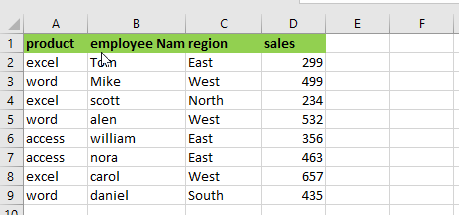We want to insert the line break in cell A5 in this example. So we double-click the cell, move the cursor to where we want the line break (after “`w`“), and hit “ALT+ENTER.” After that, we obtain a result.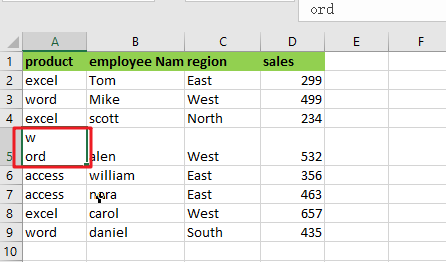In the same way, we may place line breaks in any cell. Assume we want to insert a line break after the second “e” in cell A4.

To achieve this, double-click cell A3 and move the cursor after the second “`e`,” then press Alt+Enter.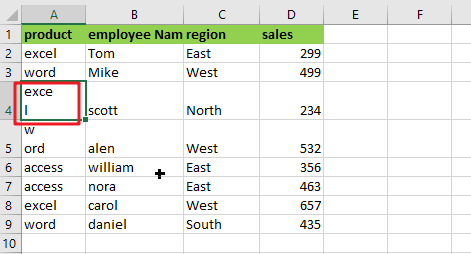We now have another example:

In cell C1, we have placed a line break after the first three digits of the mobile phone number. However, this technique becomes time-consuming and inefficient when executing the same thing in several cells. In that scenario, we employ a formula to insert a line break.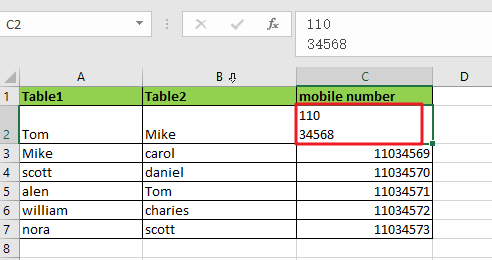## 2nd Method: With Formula

Suppose you got a task to insert line breaks in many Excel cells here we cannot utilize a keyboard shortcut. In this scenario, we must need another way to insert the line break in many Excel cells, and that way is by using a formula written as follows.

`=”text”&CHAR(10)&”text”`

For understanding, it easily lets suppose we have the following table in MS Excel, and we want to write the text from cells A2, B2, and C2 in D2 with line breaks.

We would use the concatenation operator with the CHAR function in the Formula mentioned above for inserting the line break. The ASCII code, which is a character code, is used to return the result of the CHAR function. Here are some examples to explain how this Formula works.

First, enable Wrap Text and then enter the formula based on the CHAR function:

`=A2&CHAR(10)&B2&CHAR(10)&C2`

Using the ampersand (the concatenation operator in MS Excel), we would join the text in A2, B2, and C2 in the above-written Formula after that would get the following results.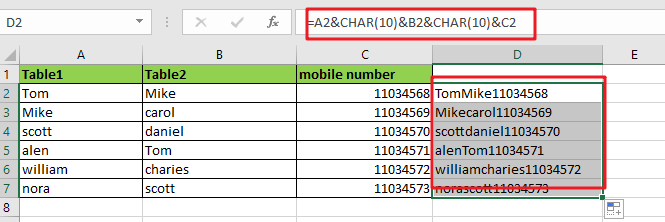The Wrap Text function is not activated by default when you insert a line break with the aid of Formula. You must need to turn it on yourself.

Select the cell with the line break in the Formula, then select the Wrap Text command on the Home tab of the Ribbon.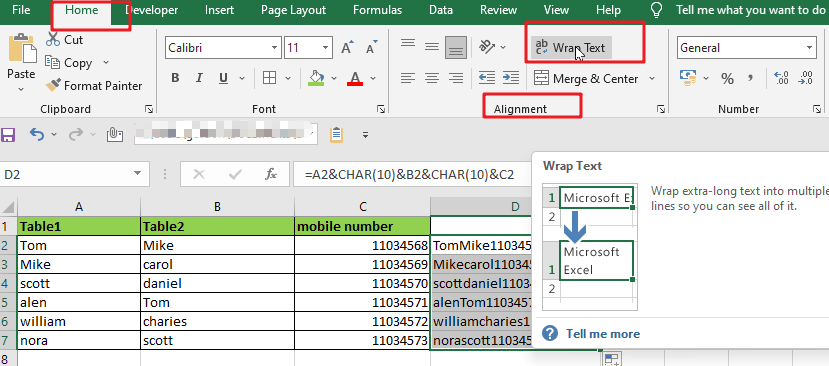Then, instead of the little square, you’ll observe a line break in the cell.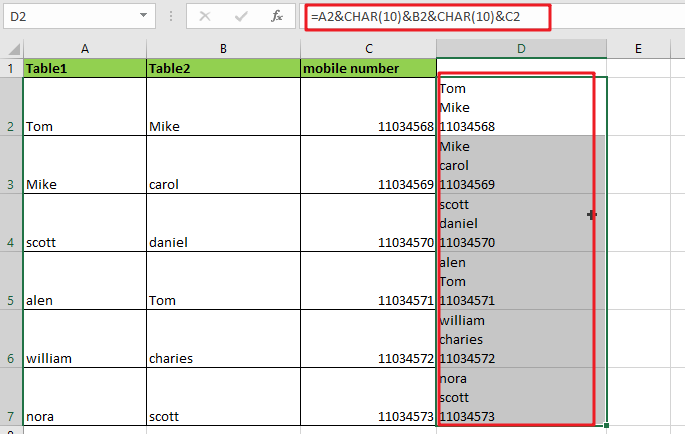### Related Functions

• Excel CHAR function
The Excel CHAR function returns the character specified by a number (ASCII Value).The CHAR function is a build-in function in Microsoft Excel and it is categorized as a Text Function. The syntax of the CHAR function is as below:=CHAR(number)….